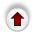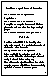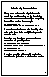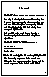## 4.1. Efficient Recursion

This section contains extra material that is not part of the course but supplements it with essential insights about recursion and its efficient use.

Recursion is such an elegant and powerful technique that a lot of effort has been invested in creating compilers that can optimize it and make its use competitive with iteration.### Iteration as a special case of recursion

The first insight is that iteration is a special case of recursion.

`        void do_loop () { do { ... } while (e); }`
is equivalent to:
`        void do_loop () { ... ; if (e) do_loop(); }`
A compiler can recognize instances of this form of recursion and turn them into loops or simple jumps. E.g.:
`        void do_loop () { start: ...; if (e) goto start; }`
Notice that this optimization also removes the space overhead associated with function calls.

### Tail Calls

The second insight concerns tail calls. A call is said to be a tail call if it is the last thing that needs to be executed in a particular invocation of the function where it occurs. For example:

`        void zig (int n) { ... ; if (e) zag(n-1); }`
The call to `zag` is a tail call because, if it happens - i.e. if `(e)` evaluates to true - then it is the last thing that needs to be executed in the current invocation of `zig`.

What it so special about tail calls? Simply this: if `zag` is the last thing to be executed in `zig`, surely `zig` won't need its local variables while `zag` is executing, and it won't need them after `zag` returns since there won't be anything left to do. Therefore, we can release the local space allocated to `zig` before calling `zag`.

Thus, tail recursive algorithms can be optimized to execute in constant space - a tail recursive algorithm is one where the recursive steps are all tail calls

### Good News & Bad News

The bad news is that often the most natural version of an algorithm is not tail recursive. Consider the factorial function:
```        int factorial(int n)
{ return (n == 0) ? 1 : n * factorial(n-1); }```
The recursive call to `factorial` is not tail recursive: the last thing that needs to be done is the multiplication, not the call. Therefore, `factorial` executes in space proportional to `n` (linear space).

The good news is that it is often not too difficult to turn a non tail-recursive algorithm into a tail-recursive one. Typically, this is done by adding extra parameters to the definition: these parameters serve to accumulate intermediate results.For example, the definition of `factorial` can be augmented with an `accumulator':

```        int factorial(int n,int accu)
{ return (n == 0) ? accu : factorial(n-1,n*accu); }```
Or we can keep the same interface as before and use an auxiliary definition:
```        int fact_aux (int n,int accu)
{ return (n == 0) ? accu : fact_aux(n-1,n*accu); }
int factorial(int n) { return fact_aux(n,1); }```
A modern optimizing compiler will turn this version into machine code equivalent to the iterative version.

How do we know that this second version is correct? We prove it by induction. You will notice that there is a strong connection between recursion and induction. They are really two aspects of the same fundamental idea.

Notice that, for n>0, fact_aux(n,a) = fact_aux(n-1,n*a). On the right-hand side of the equation, the first argument has decreased by 1. As long as n is sufficiently large, we can iterate the process:

```fact_aux(n,a) = fact_aux(n-1,n*a)
= fact_aux(n-2,(n-1)*n*a)
= fact_aux(n-3,(n-2)*(n-1)*n*a)
...
= fact_aux(n-k,(n-k+1)*...*(n-2)*(n-1)*n*a)```
in particular for k=n, we have:
```fact_aux(n,a) = fact_aux(0,1*2*...*(n-2)*(n-1)*n*a)
= 1*2*...*(n-2)*(n-1)*n*a```
because when its 1st argument is 0, fact_aux simply returns its 2nd argument. By definition of factorial:
`factorial(n) = fact_aux(n,1) = 1*2*...*(n-2)*(n-1)*n*1`
This result is precisely `n!'.

### Optimizing the Fibonacci algorithmThe fibonacci function is defined by the following equations:

• fib(0) = 1
• fib(1) = 1
• fib(n) = fib(n-2) + fib(n-1), if n>1
which we can directly implement by:
```int fib(int n)
{ return (n == 0 || n == 1) ? 1 : fib(n-2)+fib(n-1); }```
Unfortunately, there are two sources of inefficiency. Firstly, this algorithm is not tail recursive. Secondly, it spends a lot of time recomputing the same values over and over again. To wit, in order to compute fib(n):
• First we compute fib(n-2), which in particular involves computing fib(n-3).

• Then we compute fib(n-1), which requires recomputing both fib(n-2) and fib(n-3).
We can improve the algorithm as follows: we notice that the computation of fib(n-2) involves computing fib(n-3); therefore, if we could only save these two results, we could subsequently just add them together to produce fib(n-1). This the basis for our first optimization.

We are going to introduce the auxiliary function fib2 which is exactly like fib, but returns a compound value containing the two aforementioned results: i.e. fib2(n) contains both fib(n) and fib(n-1). Then, we shall write the function fib1 which computes the same value as fib, but does it more efficiently by calling fib2.

```        typedef struct { int first,second; } Pair;
Pair fib2 (int n) {
if (n == 0) { Pair p = {1,0}; return p; }
else {
Pair p1 = fib2(n-1);
Pair p2;

p2.first  = p1.first + p1.second;
p2.second = p1.first;

return p2;
}
}
int fib1 (int n) { return fib2(n).first; }```
How do we know this code is correct? First we verify the two base cases:
• fib1(0) = 1 = fib(0)
• fib1(1) = 1 = fib(1)
Then we proceed by induction, and show that for n>1
• fib2(n) = {fib(n),fib(n-1)}
• therefore: fib1(n) = fib2(n).first = fib(n)

The above improvement no longer spends time recomputing the same values. However, it is not tail recursive and consequently consumes stack space. It is possible to do better by using a bottom-up algorithm instead of a top-down algorithm. This time, we need to introduce 2 accumulators - they correspond to the pair of values of our first improvement.

```        int fib3(int n,int i,int j) { return (n==0)?i:fib3(n-1,i+j,i); }
int fib1(int n) { return fib3(n,1,0); }```
How do we know this code is correct? Again, we proceed by induction:
• fib1(n) = fib3(n,1,0) = fib3(n-k,fib(k),fib(k-1))
• in particular for k=n: fib1(n) = fib3(0,fib(n),fib(n-1)) = fib(n)

You may convince yourself that the recursive algorithm above is essentially equivalent to the following iterative version:

```        int fib1(int n)
{ int fib, fib_prev, fib_next, i;

for (fib=1, fib_prev=0, i=0;    i<n;
fib_next = fib+fib_prev,
fib_prev = fib,
fib      = fib_next,       i++);
return fib; }```